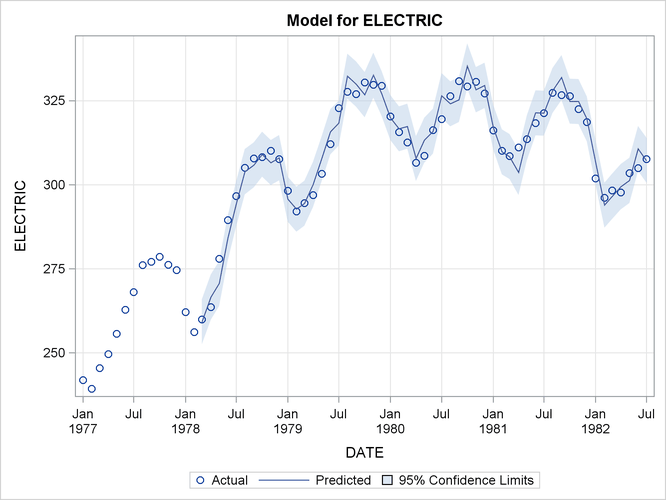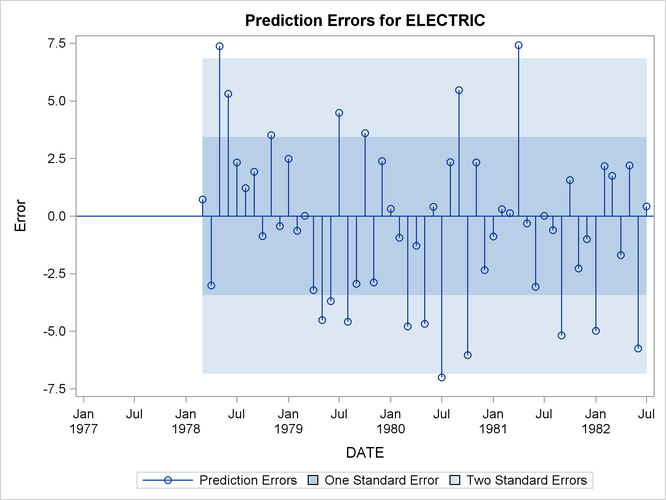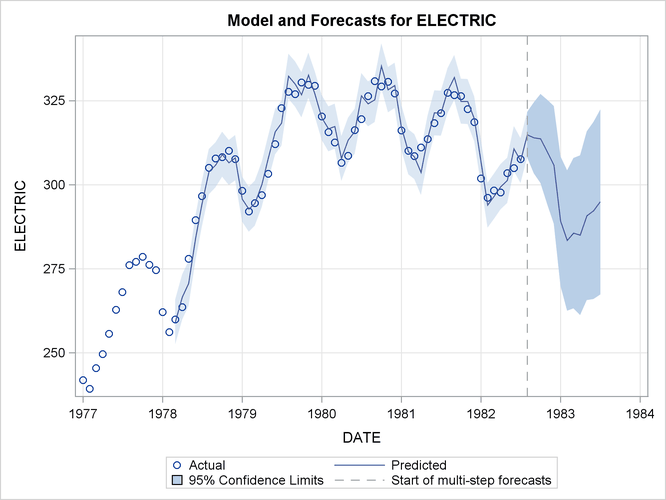# The VARMAX Procedure

### Example 35.4 Illustration of ODS Graphics

This example illustrates the use of ODS Graphics. For information about the graphics available in the VARMAX procedure, see the section ODS Graphics.

The following statements use the `SASHELP.WORKERS` data set to study the time series of electrical workers and its interaction with the series of masonry workers. The series and predict plots, the residual plot, and the forecast plot are created in Output 35.4.1 through Output 35.4.3. These are a selection of the plots created by the VARMAX procedure.

```title "Illustration of ODS Graphics";
proc varmax data=sashelp.workers plot(unpack)=(residual model forecasts);
id date interval=month;
model electric masonry / dify=(1,12) noint p=1;
run;
```

Output 35.4.1: Series and Predicted Series PlotsOutput 35.4.2: Residual PlotOutput 35.4.3: Series and Forecast Plots